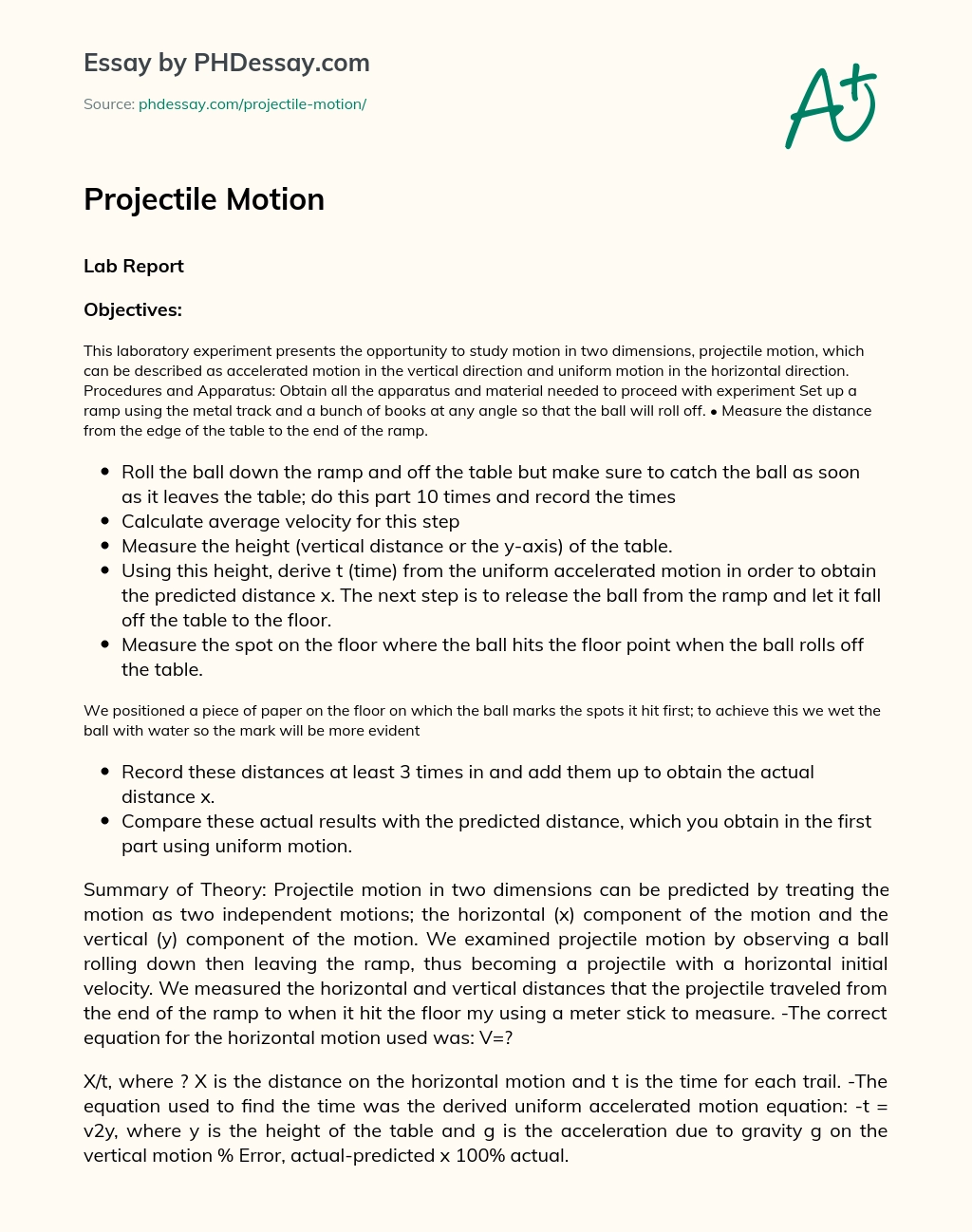Last Updated 28 Jan 2021

# Projectile Motion

Category Force, Physics
Essay type Research
Words 651 (2 pages)
Views 325

## Lab Report

### Objectives:

This laboratory experiment presents the opportunity to study motion in two dimensions, projectile motion, which can be described as accelerated motion in the vertical direction and uniform motion in the horizontal direction.

Haven’t found the relevant content? Hire a subject expert to help you with Projectile Motion

Hire writer

Procedures and Apparatus: Obtain all the apparatus and material needed to proceed with experiment

Set up a ramp using the metal track and a bunch of books at any angle so that the ball will roll off. • Measure the distance from the edge of the table to the end of the ramp.

• Roll the ball down the ramp and off the table but make sure to catch the ball as soon as it leaves the table; do this part 10 times and record the times
• Calculate average velocity for this step
• Measure the height (vertical distance or the y-axis) of the table.
• Using this height, derive t (time) from the uniform accelerated motion in order to obtain the predicted distance x. The next step is to release the ball from the ramp and let it fall off the table to the floor.
• Measure the spot on the floor where the ball hits the floor point when the ball rolls off the table.

We positioned a piece of paper on the floor on which the ball marks the spots it hit first; to achieve this we wet the ball with water so the mark will be more evident

• Record these distances at least 3 times in and add them up to obtain the actual distance x.
• Compare these actual results with the predicted distance, which you obtain in the first part using uniform motion.

Summary of Theory: Projectile motion in two dimensions can be predicted by treating the motion as two independent motions; the horizontal (x) component of the motion and the vertical (y) component of the motion. We examined projectile motion by observing a ball rolling down then leaving the ramp, thus becoming a projectile with a horizontal initial velocity. We measured the horizontal and vertical distances that the projectile traveled from the end of the ramp to when it hit the floor my using a meter stick to measure. -The correct equation for the horizontal motion used was: V=?

X/t, where ? X is the distance on the horizontal motion and t is the time for each trail. -The equation used to find the time was the derived uniform accelerated motion equation: -t = v2y, where y is the height of the table and g is the acceleration due to gravity g on the vertical motion % Error, actual-predicted x 100% actual.

## Conclusions and Observations:

Our predicted impact of (distance X) point of 47. 2cm was short by only 1cm of the actual X value of 46. 33cm. The impact points were close, so based on these results we support our predicted X value given the collected data from the experiment. I also calculated the % error and it was only 2. 14% and that again confirms our accurate result. One of the reasons for this accurate result was the technique we used to mark the point where the ball hit the floors in which we wet the ball with water so it will leave a mark on the paper place along the meter-stick. Another evidence to support our results was the height of table found from the kinematics’ equations was 76. cm while that actual measured height was 76. 3cm. As we performed the experiment we confirmed that the horizontal acceleration is always zero, but the horizontal distance that the ball covers before striking the ground does depend on initial velocity because we used uniform motion. We also leaned that Velocity in the y-direction is always zero at the beginning of the trajectory. In other words, the acceleration in the y-direction is constant, a fact that confirms the independence of vertical and horizontal motion. Through this lab, I was able to examine the affect of forces on the trajectory of a moving object.Haven’t found the relevant content? Hire a subject expert to help you with Projectile Motion

Hire writer